# How to Group Time by Hour or Minute

This post will guide you how to group time by hours intervals or group time by minute intervals in excel. How to group time by 3 hours using excel formula? How to group time by 15 minutes with formula in excel.

## Group Time by Hour or Minute

If you have a list of times in your worksheet and wonder if you want to group them into intervals such as 3 hours, one hour or 15 minutes.  How to quickly group time in excel? You can use a rounding function named as FLOOR in excel.  This function can be used to round the times based on the significance that you specified.

You can use the FLOOR function to create a generic formula as follows:

`=FLOOR(number,significance)`

Number – the number that you want to round down

Significance – the multiple of significance that you want to round a number to.

If the time is 16:00 PM and the significance value as “3:00” , then the FLOOR function should be round the time value down to the 3 hours intervals, and it will return as: 15:00 PM.

Suppose you need to group times in range A2:A6 by 3 hours, then you can write down the following formula:

`=FLOOR(A2,”3:00”)`

1# you can enter this formula into cell B2, then press Enter key.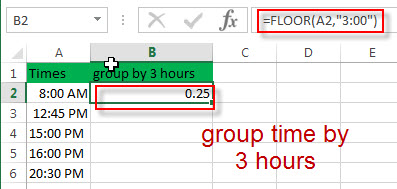2# select cell B1, right click on it, select Format Cells…, switch to Number tab, choose Time under Category list. Then click “OK” button.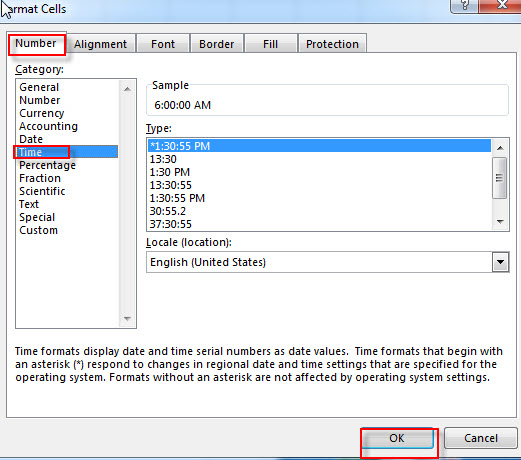3# let’s see the result.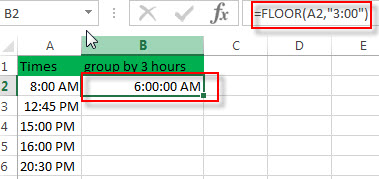4# drag the AutoFill handle down to apply this formula to other cells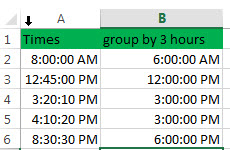If you want to group time by 15 minutes in your list of times in range A2:A6, then you use the following formula:

`=FLOOR(A2, “0.05”)`

Or

`=FLOOR(A2,TIME(0,15,0))`

You can enter this formula into Cell B1, then press Enter.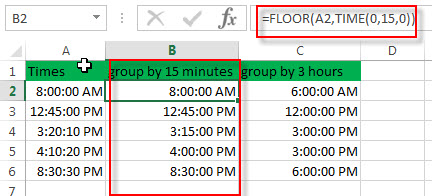## Group time with pivot table

You can also use pivot table to group times by the hour, but it only be able to group the times by 1 hour increments. It can’t group into other specific time intervals, such as: 15 minutes, or 3 hours.
1# Go to “INSERT” Tab, then click Tables -> PivotTable. Then Create PivotTable window will appear.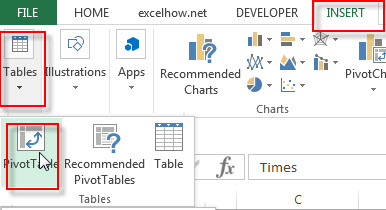2# select a table or range A1:A6, then click “OK” button.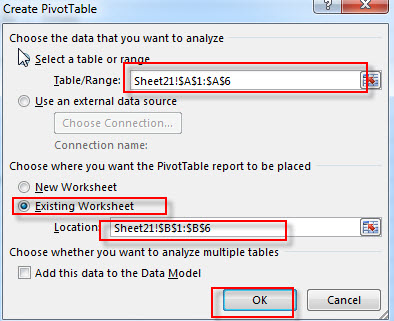3# Choose Times field to the Rows area.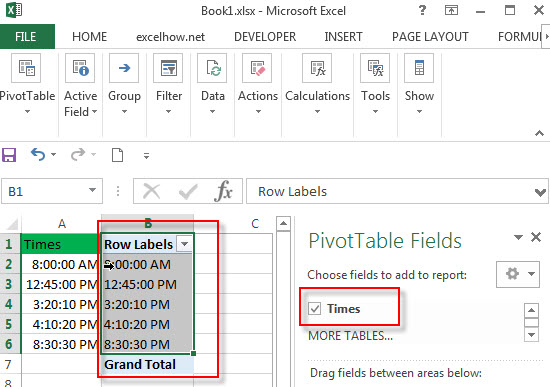4# right click on any cell in the Rows area and choose Group…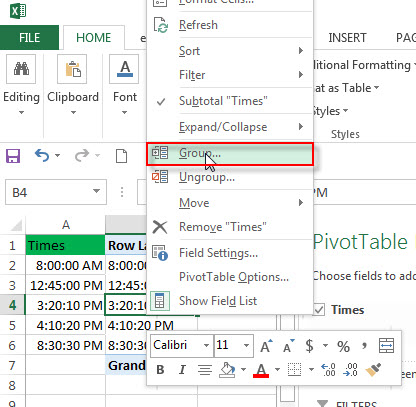5# choose Hours from Grouping window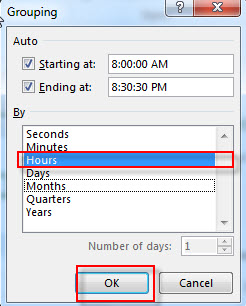6# you should see that the times have been grouped by 1 hour.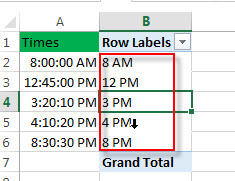### Related Functions

• Excel FLOOR function
The Excel FLOOR function returns a number rounded down to the nearest multiple of significance. So it will return a rounded number.The syntax of the FLOOR function is as below:= FLOOR (number, significance)…
• Excel TIME function
The Excel TIME function returns a date in serial number format for a particular time (the hour, minute and second).The syntax of the TIME function is as below:= TIME(hour, minute, second)…